Visualizing Missing Data with Barplot in R

• Last Updated : 27 Sep, 2021

In this article, we will discuss how to visualize missing data with barplot using R programming language.

Missing Data are those data points that are not recorded i.e not entered in the dataset. Usually, missing data are represented as NA or NaN or even an empty cell.

Dataset in use: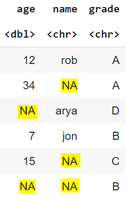In the case of larger datasets, few missing data might not affect the overall information whereas it can be a huge loss in information in the case of smaller datasets. These missing data are removed or imputed depending on the dataset. To decide how to deal with missing data we’ll first see how to visualize the missing data points.

Let us first count the total number of missing values.

Example: Counting missing values

R

 # Creating a sample dataframe using 3 vectorsage = c(12,34,NA,7,15,NA)name = c('rob',NA,"arya","jon",NA,NA)grade = c("A","A","D","B","C","B")df <- data.frame(age,name,grade) # count the total number of missing valuessum(is.na(df))

Output:

5

We can also find out how many missing values are there in each attribute/column.

Example: Count missing values in each attribute/column

R

 # Creating a sample dataframe using 3 vectorsage = c(12,34,NA,7,15,NA)name = c('rob',NA,"arya","jon",NA,NA)grade = c("A","A","D","B","C","B")df <- data.frame(age,name,grade) # count number of missing values in each# attribute/columnsapply(df, function(x) sum(is.na(x)))

Output:

2   3    0

Visualizing all missing values

Let’s first visualize the frequencies for missing and non-missing values for entire data using barplot( ) function in R.

Syntax of barplot():

barplot(x, name.args = NULL, col = ” “, main = ” ” , xlab = ” “, ylab = ” ” , beside = FALSE , horiz = TRUE …)

Parameters:

• x :  vector or matrix
• names.arg : label for each bar
• col : color for the bars
• main : title of the barplot
• xlab : label for x-axis
• ylab : label for y-axis
• beside : to specify grouped or stacked barplot
• horiz : orientation of bars (horizontal or vertical)

Example: Visualizing all missing values

R

 # Creating a sample dataframe using 3 vectorsage = c(12,34,NA,7,15,NA)name = c('rob',NA,"arya","jon",NA,NA)grade = c("A","A","D","B","C","B")df <- data.frame(age,name,grade) # converting a frequency table for missing# values to dataframefreqDf <- data.frame(table(is.na(df))) # barplot for vizualisationbarplot(freqDf\$Freq , main = "Total Missing values",xlab = "Missing Data", ylab = "Frequency",        names.arg = c("FALSE","TRUE"),col = c("#80dfff","lightgreen")) # legend for barplotlegend("topright",c("Non-Missing Values","Missing Values"),fill = c("#80dfff","lightgreen"))

Output: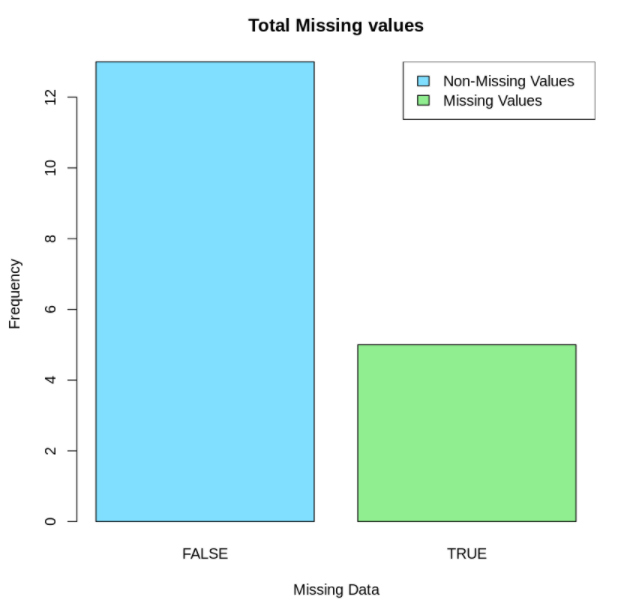Visualizing missing data for one column

For this, we select the column that we are trying to visualize and then do the needful.

Example: Visualizing missing data for one column

R

 # Creating a sample dataframe using 3 vectorsage = c(12,34,NA,7,15,NA)name = c('rob',NA,"arya","jon",NA,NA)grade = c("A","A","D","B","C","B")df <- data.frame(age,name,grade) # frequency table for missing data for 1 column,# here age column is takenfreqDf2 <- data.frame(table(is.na(df\$age))) # barplot for 1 column/featurebarplot(freqDf2\$Freq,main = "Total Missing values",xlab = "Missing Data",ylab = "Frequency",names.arg = c("FALSE","TRUE"),col = c("#ffb3b3","#99e6ff")) # legend for barplotlegend("topright",c("Non-Missing Values","Missing Values"),       fill = c("#ffb3b3","#99e6ff"))

Output: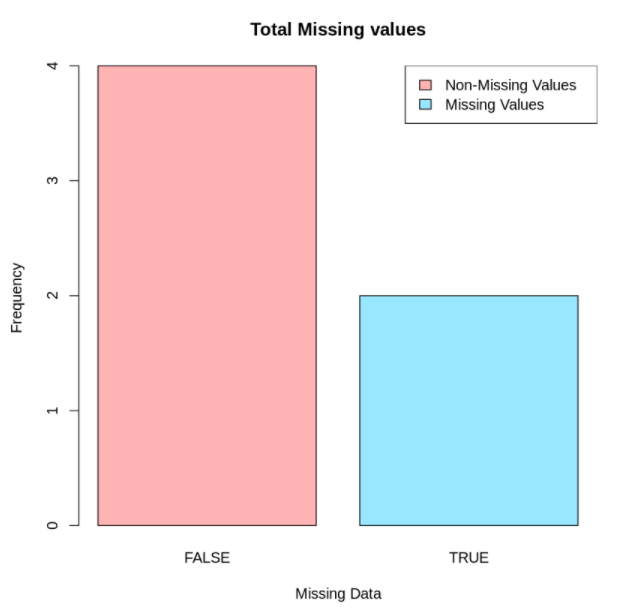Visualizing missing data for all columns

Let’s create a function to transform the dataframe to a binary TRUE/FALSE matrix and then visualize it using a barplot in R.

Example: Visualizing missing data for all columns

R

 age = c(12,34,NA,7,15,NA)name = c('rob',NA,"arya","jon",NA,NA)grade = c("A","A","D","B","C","B")df <- data.frame(age,name,grade) # function convert dataframe to binary TRUE/FALSE matrixtoBinaryMatrix <- function(df){m<-c()for(i in colnames(df)){    x<-sum(is.na(df[,i]))    # missing value count    m<-append(m,x)    # non-missing value count    m<-append(m,nrow(df)-x)} # adding column and row names to matrixa<-matrix(m,nrow=2)rownames(a)<-c("TRUE","FALSE")colnames(a)<-colnames(df) return(a)} # function callbinMat = toBinaryMatrix(df)binMat

Output:

TRUE    2     3       0
FALSE    4     3       6

Stacked barplot

The missing values can be represented in contrast with the values present using a stacked barplot.

Example: Stacked barplot

R

 age = c(12,34,NA,7,15,NA)name = c('rob',NA,"arya","jon",NA,NA)grade = c("A","A","D","B","C","B")df <- data.frame(age,name,grade) # stacked barplot for missing data in all columnsbarplot(binMat,main = "Missing values in all features",xlab = "Frequency",col = c("#4dffd2","#ff9999")) # legend for barplotlegend("bottomright",c("Missing values","Non-Missing values"),fill = c("#4dffd2","#ff9999"))

Output: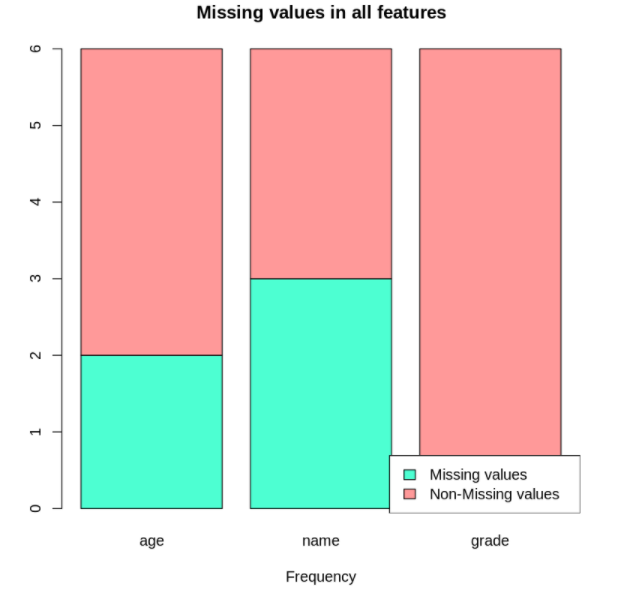Grouped barplot

Another visualization that can be helpful is a grouped barplot.

Example: Grouped barplot

R

 age = c(12,34,NA,7,15,NA)name = c('rob',NA,"arya","jon",NA,NA)grade = c("A","A","D","B","C","B")df <- data.frame(age,name,grade) # grouped barplot for missing data in all columnsbarplot(binMat,main = "Missing values in all features",xlab = "Frequency",col = c("#ffff99","#33bbff"),beside=TRUE,horiz = TRUE) # legend for barplotlegend("right",c("Missing values","Non-Missing values"),fill = c("#ffff99","#33bbff"))

Output: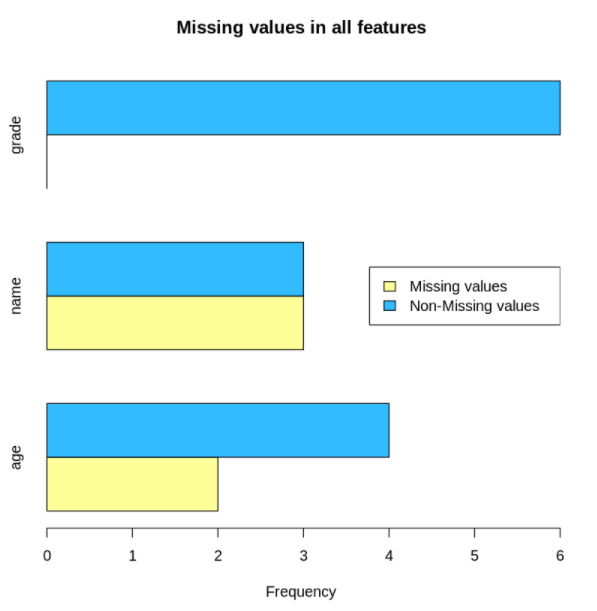My Personal Notes arrow_drop_up